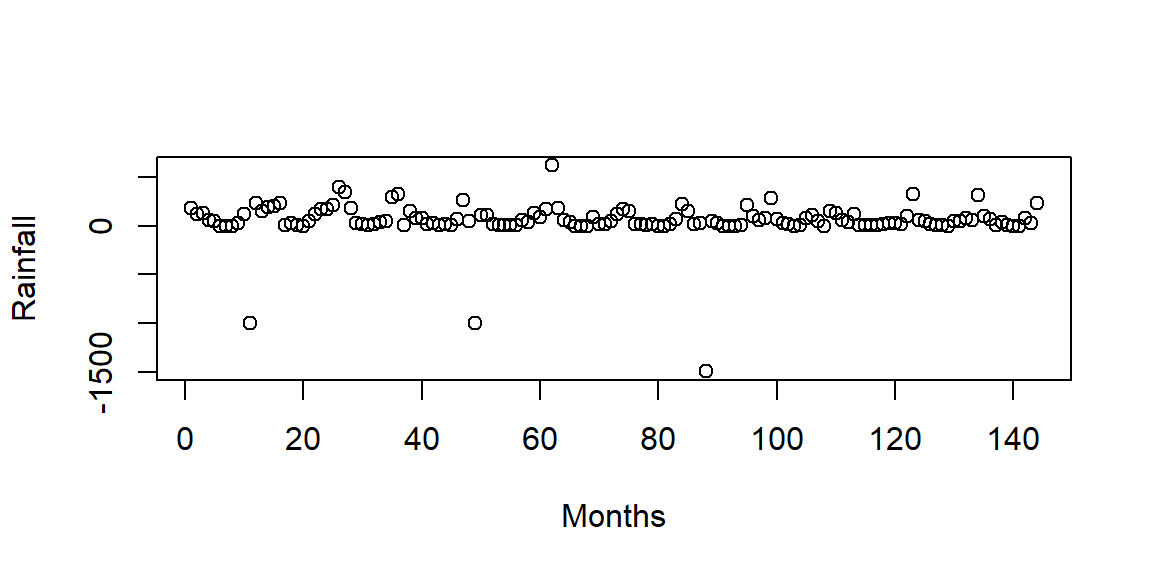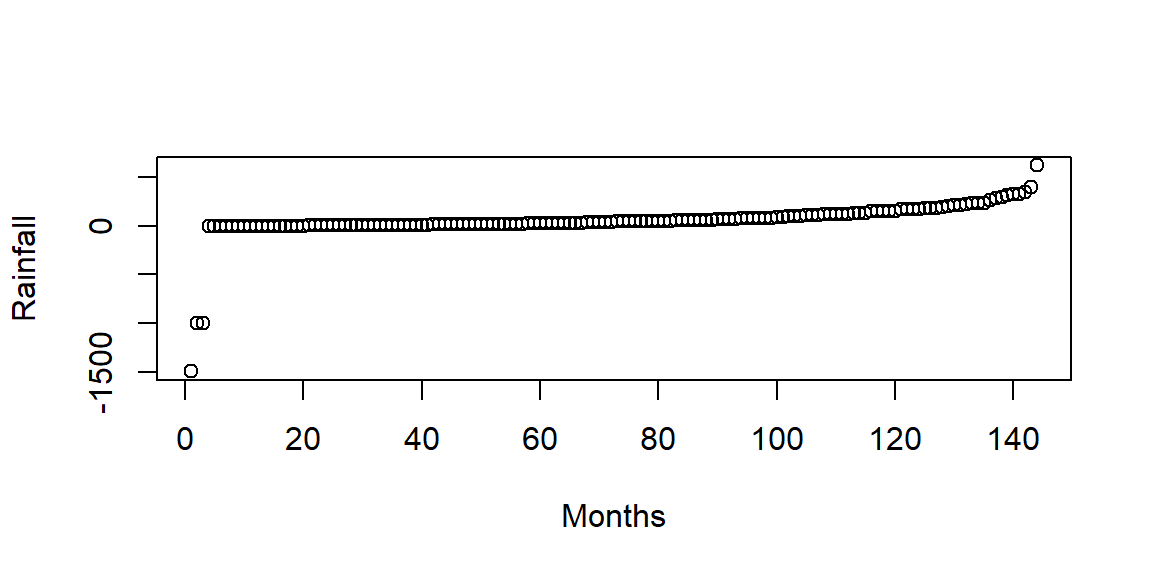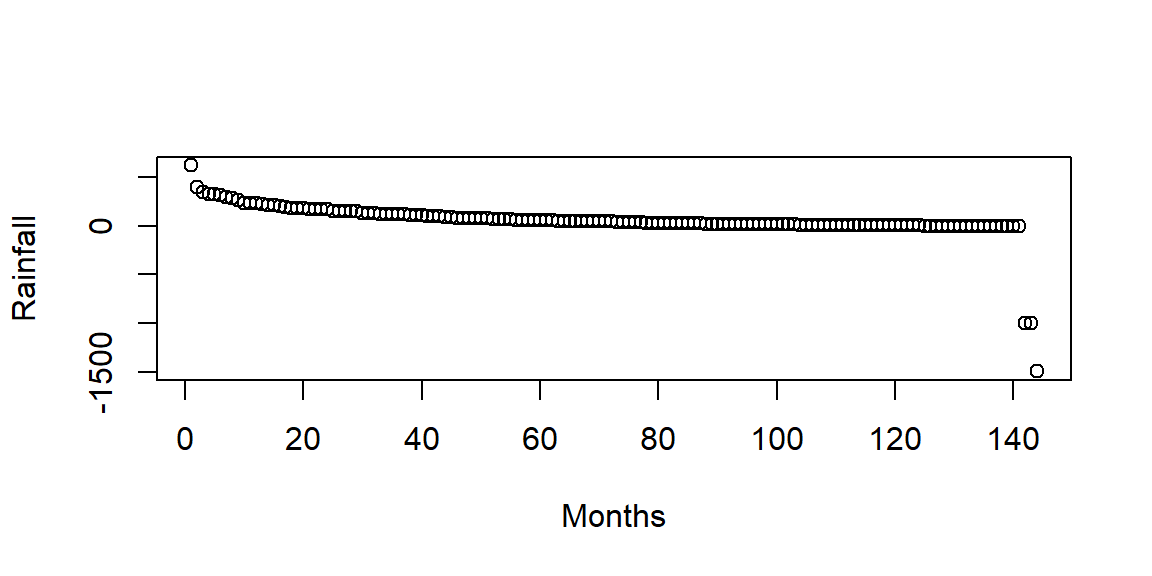### Learning objectives

• Learn how to preprocess data in R for analysis and plotting

### Content

• Data types in R: vector, matrix, dataframe, factor, character
• Read data from multiple folders
• Replace/remove missing values
• Manipulate subset of dataframe
• Calculate statistics by group
• Row/column-wise statistics
• Order dataframe values
• Reshape dataframe
• Randomly split data
• Standardize and normalize data
• For loop and if/else condition
• Join data with common column

### Data/code for this tutorial

#web link
url.data = 'https://raw.githubusercontent.com/szalam/R-tutorial/master/R_tutorial_part2_data.zip'

setwd('C:/sarfaraz/Project_R_tutorials/R-tutorial/R_beginner_part2_files/')

#unzip data
unzip(zipfile='R_tutorial_part2_data.zip')

### Data types in R

Vector: Sequence of data. Can be told as 1-dimensional data.

#Vector
v1 = c(1,2,3,4)
v2 = c('A', 'B', 'C', 'D')

print(v1)
##  1 2 3 4
print(v2)
##  "A" "B" "C" "D"

Matrix: 2-dimensional data with row and columns. Data type (or class) of all the matrix element should be same (numeric, character)

#Matrix. All values are numeric
m = matrix(1:10, nrow = 5, ncol = 2)
m
##      [,1] [,2]
## [1,]    1    6
## [2,]    2    7
## [3,]    3    8
## [4,]    4    9
## [5,]    5   10

Dataframe: 2-dimensional data with row and columns. Data type of different columns can be different. One column can be numeric and another can be character.

#Dataframe. Column 1 and 2 numeric, and column 2 character
c1 = c(1:5)
c2 = c(11:15)
c3 = c('A', 'B', 'C', 'D', 'E')
df = data.frame(c1, c2, c3)

List: Similar to 3-dimensional data. For example, a list with two objects (or lists) can have dataframe in the first list and vector in the second list. It can store different types of objects in different list.

#Lists
l = list()
l[] = df
l[] = v1
str(l)
## List of 2
##  $:'data.frame': 5 obs. of 3 variables: ## ..$ c1: int [1:5] 1 2 3 4 5
##   ..$c2: int [1:5] 11 12 13 14 15 ## ..$ c3: chr [1:5] "A" "B" "C" "D" ...
##  $: num [1:4] 1 2 3 4 Factor: Used to categorize data. Can be integer or character. Factor is very usefull during categorical plots. setwd('C:/sarfaraz/Project_R_tutorials/R-tutorial/R_beginner_part2_files/data1/') df = read.csv('rainfall_data.csv') head(df) ## Date Month Year loc_A loc_B loc_C ## 1 1/15/1981 1 1981 188.682179 83.57845383 164.6300596 ## 2 2/15/1981 2 1981 83.090653 42.52718642 46.4600436 ## 3 3/15/1981 3 1981 136.694246 99.88195830 130.9438164 ## 4 4/15/1981 4 1981 40.223348 NA 37.0420120 ## 5 5/15/1981 5 1981 46.528754 13.56783115 18.1241447 ## 6 6/15/1981 6 1981 4.081162 0.01092273 0.1125636 #lets plot year vs rainfal in loc_A in the original dataframe 'df' df.mod = df[!is.na(df$loc_A),]
#plot(df.mod$Year,df.mod$loc_A)

#convert the Year column of df into factor
df.mod2 = df.mod
df.mod2$Year = as.factor(df.mod2$Year)
# plot(df.mod2$Year,df.mod2$loc_A)

### Read data from multiple folders

A commonly used technique to read csv/txt files from different folders is shown below. Here, we read one .csv file from folder “data1”" and 1 .txt file from folder “data2”.

fold1 = 'C:/sarfaraz/Project_R_tutorials/R-tutorial/R_beginner_part2_files/data1/'
fold2 = 'C:/sarfaraz/Project_R_tutorials/R-tutorial/R_beginner_part2_files/data2/'

df2 = read.table(paste0(fold2,'d1.txt'),fill =T)

### Remove/replace missing values

Here we read a csv file with missing data (which is generally read as “NA” in R). Then remove/replace NA values.

#set working directory
setwd('C:/sarfaraz/Project_R_tutorials/R-tutorial/R_beginner_part2_files/data1')

summary(df)
##      Date               Month            Year          loc_A
##  Length:144         Min.   : 1.00   Min.   :1981   Min.   :  0.0393
##  Class :character   1st Qu.: 3.75   1st Qu.:1984   1st Qu.: 11.8359
##  Mode  :character   Median : 6.50   Median :1986   Median : 42.6371
##                     Mean   : 6.50   Mean   :1986   Mean   : 73.8314
##                     3rd Qu.: 9.25   3rd Qu.:1989   3rd Qu.:112.1323
##                     Max.   :12.00   Max.   :1992   Max.   :416.9089
##                                                    NA's   :3
##      loc_B               loc_C
##  Min.   :  0.01092   Min.   :  0.0719
##  1st Qu.:  2.93055   1st Qu.:  5.6136
##  Median : 17.68898   Median : 27.4605
##  Mean   : 34.57416   Mean   : 55.3813
##  3rd Qu.: 50.15615   3rd Qu.: 80.5734
##  Max.   :245.07228   Max.   :342.1296
##  NA's   :3           NA's   :2
#calculate mean
mean(df$loc_A) ##  NA mean(df$loc_A, na.rm = T)
##  73.83143
#find ids of NAs for loc_A, loc_B, loc_C
id.na.a = which(is.na(df$loc_A)==T) id.na.a = which(is.na(df$loc_A)==T)
id.na.a = which(is.na(df$loc_A)==T) #replace NA with -999 df[id.na.a,4] = -999 #remove rows with NA values in loc_A df2 = df[-id.na.a,] #another way to remove rows with NA in a single line df3 = df[!is.na(df$loc_A),]

Similar to rows, specific column can also be removed from the dataframe

#removing column 2 from df
df.rm = df[,-2]

#removinb column 2 and 3
df.rm.2 = df[,c(-2,-3)]

### Replace character/numeric

Replacing character/numeric value is a neccesary is skill. For example, a data may have spelling mistake (“Californiaa”" instread of “California”). Here, we will learn how to replace character/numreic values.

#set working directory
setwd('C:/sarfaraz/Project_R_tutorials/R-tutorial/R_beginner_part2_files/data1')

#read data and check unique Regions
unique(df.tmp$Region) ##  "California" "Ohio" "Nevada" "Californiaa" #looks like there is a spelling mistake. "Californiaa" should be "California". Lets correct it df.tmp[df.tmp$Region == 'Californiaa',] = 'California'
unique(df.tmp$Region) ##  "California" "Ohio" "Nevada" Using ifelse function can be an efficient way to do the replacing job. #here we will replace Region Ohio with Washington. The code replace where region is Ohio, otherwise keep as it is. df.tmp$Region = ifelse(df.tmp$Region == "Ohio", "Washington", df.tmp$Region)
unique(df.tmp$Region) ##  "California" "Washington" "Nevada" The method I showed above are also applicable for numeric values or signs. For example, you might want to replace numeric values, or signs like “#” or “?” or even blank spaces " ". The above codes are extremely useful for data cleaning and preparation for analysis. ### Manipulate subset of dataframe I will show three ways to select part of a dataframe that follow user-specified condition. Let us separate data with months 1,3 and 7 #method 1: identify the indices, then separate id.tmp = which(df$Month == 1 | df$Month == 3 | df$Month == 7)
df.new1 = df[id.tmp,]

#method 2: using %in%
mon.tmp = c(1,3,7)
df.new2 = df[df$Month %in% mon.tmp,] #method 3: using filter funtion of the library dplyr. Call dplyr library first library(dplyr) # if you don't have the library than install using install.packages('dplyr') ## ## Attaching package: 'dplyr' ## The following objects are masked from 'package:stats': ## ## filter, lag ## The following objects are masked from 'package:base': ## ## intersect, setdiff, setequal, union df.new3 = filter(df, Month == c(1,3,7)) #to select subset within a range (2 to 8) df.new4 = filter(df, Month <=8 & Month >=2) Let us multiply 1.5 with all the rainfall in month 2 and 4 that occurred in loc_A #identify the indices of rows with month 2 and 4 id.tmp = which(df$Month == 2 | df$Month == 4) #multiply 1.5 with the loc_A column having row id as id.tmp df[id.tmp,4] = df[id.tmp,4] * 1.5 ### Calculate statistics by group Let us calculate mean rainfall in all the January months (month = 1). #using aggregate function df.gr1 = aggregate(df[,c(4,5,6)],by = list(df[,2]),FUN = mean,na.rm = T) head(df.gr1) ## Group.1 loc_A loc_B loc_C ## 1 1 26.42661 59.006145 85.862767 ## 2 2 202.93061 65.025331 104.953206 ## 3 3 168.03628 91.019927 136.666929 ## 4 4 -49.59144 28.554692 45.024609 ## 5 5 36.71198 10.733013 19.447401 ## 6 6 17.97584 4.148091 7.806769 ### Row/column-wise statistics Calculate mean value of all rows/columns #calculate mean along selected columns (here, column 4, 5 and 6) df.gr2.mean = apply(df[,c(4,5,6)], 2, mean,na.rm = T) # here 2 means column-wise #also calculate column-wise standard deviation df.gr2.sd = apply(df[,c(4,5,6)], 2, sd,na.rm=T) #calculate row-wise mean in selected columns (here, column 4, 5 and 6) df.gr3 = apply(df[,c(4,5,6)], 1, mean,na.rm = T) # here 1 indicate row # we can also do this using colMeans() and rowMeans() functions df.gr2_2 = colMeans(df[,c(4,5,6)], na.rm =T) df.gr2_2 ## loc_A loc_B loc_C ## 55.74019 34.57416 55.38134 df.gr3_2 = rowMeans(df[,c(4,5,6)], na.rm =T) ### Order dataframe values Let us sort the datframe in ascending/descending order #sort in ascending order df.tmp = df[order(df$loc_A),]
head(df.tmp)
##           Date Month Year         loc_A      loc_B        loc_C
## 88   4/15/1988     4 1988 -1498.5000000 60.2571635  77.04603099
## 11  11/15/1981    11 1981  -999.0000000 50.1490205 162.31109130
## 49   1/15/1985     1 1985  -999.0000000 28.2914828  26.01976957
## 8    8/15/1981     8 1981     0.0393286  0.5085948   0.16317945
## 103  7/15/1989     7 1989     0.1020899  0.2536141   0.07192623
## 68   8/15/1986     8 1986     0.1706050  0.8371909   0.50209573
#sort in descending order
df.tmp2 = df[order(-df$loc_A),] #plot plot(df$loc_A, ylab = 'Rainfall', xlab = 'Months')
plot(df.tmp$loc_A, ylab = 'Rainfall', xlab = 'Months') plot(df.tmp2$loc_A, ylab = 'Rainfall', xlab = 'Months')### Reshape dataframe

Dataframe can be reshaped in different ways. I am showing few of them below,

#transpose
tr = t(df[,c(4,5,6)])
#after transpose dataframe became matrix. to convert to dataframe
df.t = as.data.frame(tr)

Melt dataframe to obtain unique ids. This is specially usefull while using ggplot

#melt dataframe. This creates unique ids. we need 'reshape2' package for melt function
library(reshape2)
df.m = melt(df, id = c('Date','Year','Month'))
#all rainfall is moved to a single column 'value' and location names to column 'variable'
head(df.m)
##        Date Year Month variable      value
## 1 1/15/1981 1981     1    loc_A 188.682179
## 2 2/15/1981 1981     2    loc_A 124.635979
## 3 3/15/1981 1981     3    loc_A 136.694246
## 4 4/15/1981 1981     4    loc_A  60.335022
## 5 5/15/1981 1981     5    loc_A  46.528754
## 6 6/15/1981 1981     6    loc_A   4.081162

### Randomly splitting data

A lot of application requires data to be randomly splitted. One of which is to train a model and another for validation. Here, we split the dataframe into two groups where the first group has 60% data and the second has 40%.

id = sample(2, nrow(df), replace = TRUE, prob = c(0.6, 0.4))

#separate rows where id == 1 and id == 2 in two variables
grp1 = df[id==1,]
grp2 = df[id==2,]

dim(df)
##  144   6
dim(grp1)
##  86  6
dim(grp2)
##  58  6

### Standardize and normalize data

Standardization: Removing the mean and variability from a data. Mean becomes 0 and standard deviation 1. Normalization: There are different ways to normalize a data. Common way is to subtract mean and divide by the range (maximum - minimum).

#standardization of rainfall in location A
scale.df= scale(df$loc_A) #normalize rainfall in location A nor.locA = (df$loc_A - min(df$loc_A, na.rm = T))/(max(df$loc_A, na.rm = T) - min(df\$loc_A, na.rm = T))

### For loop and if/else

#change working directory to the data location
setwd('C:/sarfaraz/Project_R_tutorials/R-tutorial/R_beginner_part2_files/data2/')

#list down files with .txt extension
files = list.files(pattern='.txt')

#total files listed
length(files)
##  3

Read files in loop and apply if/else condition. I am creating two examples for this

#### Example 1:

Read texts in loop and print texts if missing value is more than or equal 9 in a column

#change working directory to the data location
setwd('C:/sarfaraz/Project_R_tutorials/R-tutorial/R_beginner_part2_files/data2/')

#for loop
for(i in 1:length(files)){ # iterate 3 times

#read txt file. fill = T means the missing value is filled

if(length(which(is.na(df.tmp[,4])==T))<=9){
print(paste0('i=',i,' Note:less than or equal 9 missing values'))
}else{
print(paste0('i=',i,' Note:more than 9 missing values'))
}
}
##  "i=1 Note:less than or equal 9 missing values"
##  "i=2 Note:more than 9 missing values"
##  "i=3 Note:less than or equal 9 missing values"

#### Example 2:

Same as the previous example, but skipping a loop when missing value greater than 9

#change working directory to the data location
setwd('C:/sarfaraz/Project_R_tutorials/R-tutorial/R_beginner_part2_files/data2/')

#for loop
for(i in 1:length(files)){ # iterate 3 times

#read txt file. fill = T means the missing value is filled
if(length(which(is.na(df.tmp[,4])==T))<=9){
print(paste0('i=',i,' Note:less than or equal 9 missing values'))
}else{
next()
}
}
##  "i=1 Note:less than or equal 9 missing values"
##  "i=3 Note:less than or equal 9 missing values"

### Join data with common column

Let us read two sets of data that have date as a common axis. We join two dataframes using full_join command of dplyr library. Here, I show joining based on date, but this can be used for joining based on different types of values (like numeric and factor)

setwd('C:/sarfaraz/Project_R_tutorials/R-tutorial/R_beginner_part2_files/data2/')

head(df.new.join)
##        Date Month.x Year.x      loc_A Month.y Year.y       loc_B
## 6 6/15/1981       6   1981   4.081162       6   1981  0.01092273